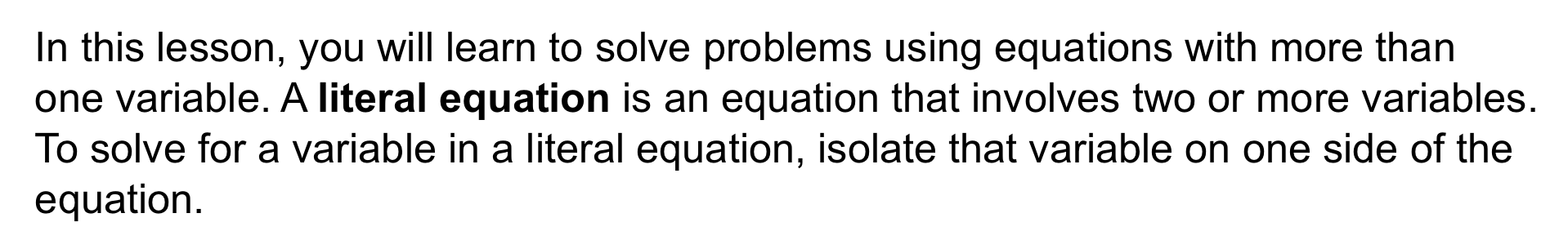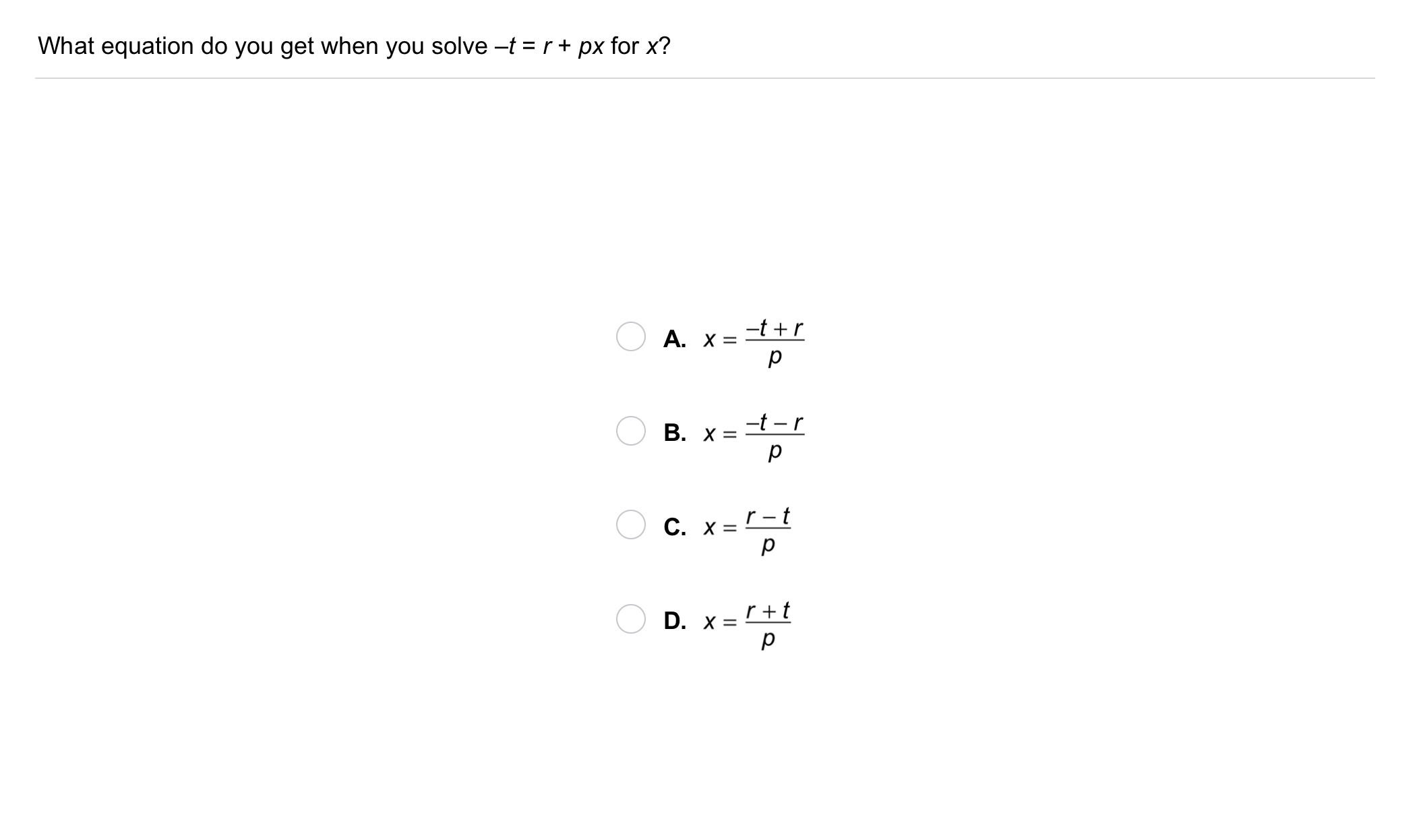Algebra 1 2-5 Guided Practice: Literal Equations and Formulas
starstarstarstarstarstarstarstarstarstar
by Matthew Richardson
| 15 Questions1
2
3
1
10
Solve It! You are ordering pizzas and sandwiches. You have a budget of \$80. How many sandwiches can you buy if you buy 4 pizzas?
Enter only a number.
2
10
Enter only a number.
3
104
5
6
4
10
Take Note: Define literal equation.
5
5
Take Note: Provide an example of a literal equation.
6
10
Take Note: How can you solve for a variable in a literal equation?
7
40
Problem 1 Got It? Solve the literal equation for m. Show your work on the canvas.

8
10
Problem 1 Got It? What is the value of m for the given value of n?
Enter only a number.
9
10
Problem 1 Got It? What is the value of m for the given value of n?
Enter only a number.
10
10
Problem 1 Got It? What is the value of m for the given value of n?
Enter only a number.11
11
10
Problem 2 Got It?
A
B
C
D12
12
10
Take Note: Define formula.13
13
10
Problem 3 Got It?
A
B
C
D14
14
10
Problem 4 Got It? Pacific gray whales migrate annually from the waters near Alaska to the waters near Baja California, Mexico, and back. The whales travel a distance of about 5000 mi each way at an average rate of 91 mi per day. About how many days does it take the whales to migrate one way?
A
B
C
D15
15
10
Take Note: Summarize the mathematical content of this lesson. What topics, ideas, and vocabulary were introduced?